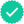#### Related questions#### Concept explainers

Question

Suppose x is a real number and x is not equal to zero. Prove that if ((x^1/3)+5)/((x^2)+6)=1/x, then x is not equal to 8.

Expert SolutionKnowledge Booster••